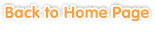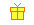Sample questions are shown below. Just click to start!• Chapter 1
Rational Numbers
•
Rational numbers on the Number line•••
Multiplying and Dividing Rational Numbers•• Chapter 2
Linear Equations and Problem Solving
•
Variables and Expressions•
Simplify the Expression••
Solving Simple Equations•
Solving Linear Equations•• Chapter 3
Systems of Linear Equations
•
Linear Equation Systems in Two Variables••
Solving Systems by Graphing•
Solving Word Problems• Chapter 4
Inequalities
•
Introduction to Inequalities••
Solving Word Problems Involving Inequalities•
Systems of Inequalities in One Variable• Chapter 5
Geometry
•
Solid Figures••
Angles• Chapter 6• Chapter 7• Chapter 8
Triangles
••
Internal Angle Sum and the Combination of Polygons• Chapter 9